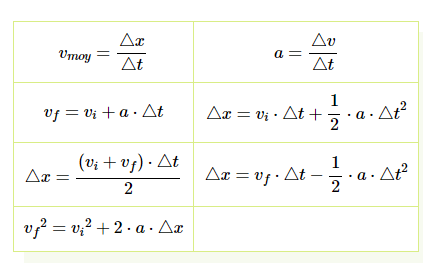# Help Zone

### Student Question

I have a physics problem. I can't seem to find a way to solve it.

A cart of mass 500 kg is rolling with a speed of 5 m/s on a railway track. There is very little friction between the tracks and the cart.  You apply a constant force on the cart in the direction opposite to its velocity.  From the moment you start applying the force, the cart moves 30 m before coming to a stop.  What was the approximate magnitude of the force you applied?

Physics{t c="richEditor.description.title"} {t c="richEditor.description.paragraphMenu"} {t c="richEditor.description.inlineMenu"} {t c="richEditor.description.embed"}

## Explanations (1)

•Explanation from AlloprofExplanation from Alloprof

This Explanation was submitted by a member of the Alloprof team.

Hi GammaRabbit8261,

Thank you for your question!

First off, you'll need to identify the information given, what you're looking for and the correct formulas to find it. From the problem, we know that the object has a mass of 500kg, an initial speed of 5 m/s, when a force is applied in the opposite direction of the movement, it moves 30 m before stopping. We are looking for the force applied in this case.

We know that we'll need to find a force, thus we know that we will need to find an acceleration. According to Newton's second law, we know that a force is equal to the product of the mass and the acceleration, F = ma. That means that we need to find the acceleration.

To find this acceleration, we need to use the information that is given to us. We know the initial/final speed and the movement of the cart. We can use one of these formulas to find the acceleration:Once you have the acceleration, you'll simply need to use the mass and Newton's second law to find the force!

I hope that helps and let us know if we can help you with anything else!

Anthony B.